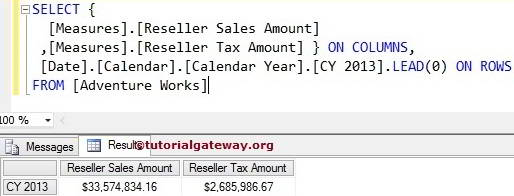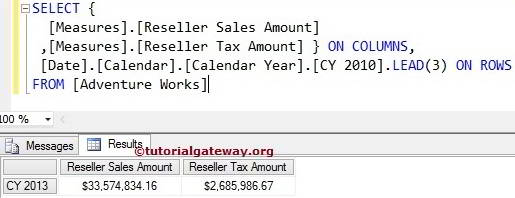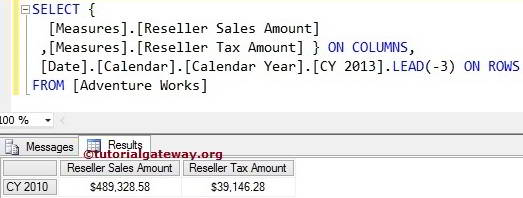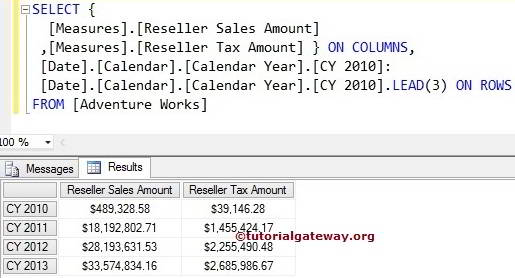The MDX Lead function is used to navigate between same level members and returns the members at the specified position. For example, If you want to navigate to a particular member, then we can use this LEAD function.

The basic syntax of the LEAD Function in Multidimensional Expression is as shown below:

`Member_Expression.LEAD (Member_Position)`

Member_Expression: Any Multidimensional Expression that returns valid Member.

Member_Position: Please specify the position of a member you want to Navigate.

• If we use Zero as the Member_Position, the MDX Lead Function will write the same Member_Expression that we mentioned before Lead function.
• If we use Positive Value, the Lead Function will move forward to the specified value and returns the Member_Expression at that position.
• If we use Negative Value, the Lead Function will move Backwards to the specified value and returns the Member_Expression at that position.

The Lead Function used to navigate between the same Members level. For example, If we mention a City member as Member_Expression, the Lead function will navigate between the remaining Cities. It will not go Level Up (State – Province) or Level down (Postal Code).

How to write a MDX Lead function to navigate both forward and backward with examples?. For this, we are going to use the below-shown data.

## MDX Lead Function with Zero

In this example, we will show you, What happens when we use Zero value for the Lead Function. The following query will return the Reseller Sales amount and Tax amount of the Calendar Year 2013 itself.

TIP: In MDX, Lag Function with a Negative value is Equal to the Lead function with a Positive Value.

```SELECT {
[Measures].[Reseller Sales Amount]
,[Measures].[Reseller Tax Amount] } ON COLUMNS,## MDX Lead Function with Positive Value

In this example, we will show you, What happens when we use a Positive integer value in the Lead Function. The following query will display the Reseller Sales and Tax amount of the Calendar Year 2013 because the Lead function with positive value (3) will move forward 3 years from the given year (2010 + 3 = 2013)

```SELECT {
[Measures].[Reseller Sales Amount]
,[Measures].[Reseller Tax Amount] } ON COLUMNS,## Lead Function with Negative Value

What happens when we use a Negative integer value for the MDX Lead Function?. The following query will return the Reseller Sales amount and Tax amount of the Calendar Year 2010 because the Lead function with a negative value (-3) will move back 3 years from the given year (2013 – 3 = 2010)

```SELECT {
[Measures].[Reseller Sales Amount]
,[Measures].[Reseller Tax Amount] } ON COLUMNS,## Finding Range Using MDX Lead Function

This example shows you how to find the Range using the Lead function. The following query will return the Reseller Sales and Tax amount from Calendar Year 2010 to 2013 because we used the range symbol (:) between [CY 2010] and [CY 2010].LEAD (3).

```SELECT {
[Measures].[Reseller Sales Amount]
,[Measures].[Reseller Tax Amount] } ON COLUMNS,
[Date].[Calendar].[Calendar Year].[CY 2010]:If you want to find the range by moving backward, then use the following MDX query.

```SELECT {
[Measures].[Reseller Sales Amount]
,[Measures].[Reseller Tax Amount] } ON COLUMNS,
[Date].[Calendar].[Calendar Year].[CY 2013]:Suresh is the founder of TutorialGateway and a freelance software developer. He specialized in Designing and Developing Windows and Web applications. The experience he gained in Programming and BI integration, and reporting tools translates into this blog. You can find him on Facebook or Twitter.